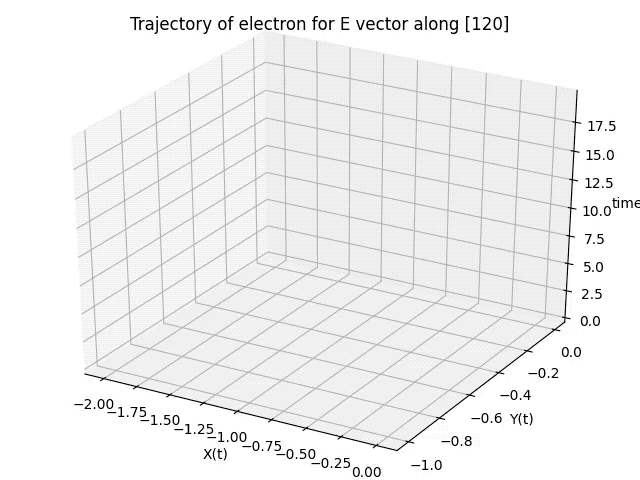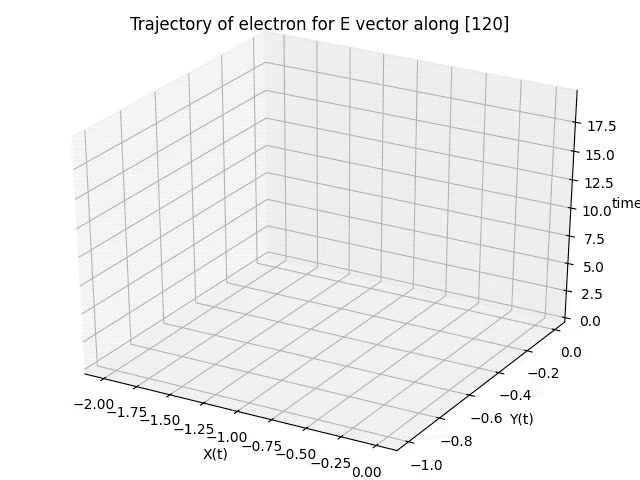# 3D Trajectory Animated using Matplotlib (Python)

Based on a lot of useful references especially (https://gist.github.com/neale/e32b1f16a43bfdc0608f45a504df5a84) here I share my code to plot the animated trajectory of a particle (in this case electron) using a 3D line/scatter chart.

In my last two blog posts I have already shown how to create an animated 2D line/scatter plot and how to plot a 3D chart.
Here we combine the two codes.

If you just want a line chart then use the following:

### CODE

```import numpy as np
import matplotlib.pyplot as plt
import matplotlib.animation as animation
from mpl_toolkits.mplot3d import Axes3D

# References
# https://gist.github.com/neale/e32b1f16a43bfdc0608f45a504df5a84
# https://towardsdatascience.com/animations-with-matplotlib-d96375c5442c
# https://riptutorial.com/matplotlib/example/23558/basic-animation-with-funcanimation

# ANIMATION FUNCTION
def func(num, dataSet, line):
# NOTE: there is no .set_data() for 3 dim data...
line.set_data(dataSet[0:2, :num])
line.set_3d_properties(dataSet[2, :num])
return line

# THE DATA POINTS
t = np.arange(0,20,0.2) # This would be the z-axis ('t' means time here)
x = np.cos(t)-1
y = 1/2*(np.cos(2*t)-1)
dataSet = np.array([x, y, t])
numDataPoints = len(t)

# GET SOME MATPLOTLIB OBJECTS
fig = plt.figure()
ax = Axes3D(fig)

# NOTE: Can't pass empty arrays into 3d version of plot()
line = plt.plot(dataSet, dataSet, dataSet, lw=2, c='g') # For line plot

# AXES PROPERTIES]
# ax.set_xlim3d([limit0, limit1])
ax.set_xlabel('X(t)')
ax.set_ylabel('Y(t)')
ax.set_zlabel('time')
ax.set_title('Trajectory of electron for E vector along ')

# Creating the Animation object
line_ani = animation.FuncAnimation(fig, func, frames=numDataPoints, fargs=(dataSet,line), interval=50, blit=False)
#line_ani.save(r'AnimationNew.mp4')

plt.show()

```

### OUTPUTFor a combination of Scatter and Line chart use the following code

### CODE

```import numpy as np
import matplotlib.pyplot as plt
import matplotlib.animation as animation
from mpl_toolkits.mplot3d import Axes3D

# References
# https://gist.github.com/neale/e32b1f16a43bfdc0608f45a504df5a84
# https://towardsdatascience.com/animations-with-matplotlib-d96375c5442c
# https://riptutorial.com/matplotlib/example/23558/basic-animation-with-funcanimation

# ANIMATION FUNCTION
def func(num, dataSet, line, redDots):
# NOTE: there is no .set_data() for 3 dim data...
line.set_data(dataSet[0:2, :num])
line.set_3d_properties(dataSet[2, :num])
redDots.set_data(dataSet[0:2, :num])
redDots.set_3d_properties(dataSet[2, :num])
return line

# THE DATA POINTS
t = np.arange(0,20,0.2) # This would be the z-axis ('t' means time here)
x = np.cos(t)-1
y = 1/2*(np.cos(2*t)-1)
dataSet = np.array([x, y, t])
numDataPoints = len(t)

# GET SOME MATPLOTLIB OBJECTS
fig = plt.figure()
ax = Axes3D(fig)
redDots = plt.plot(dataSet, dataSet, dataSet, lw=2, c='r', marker='o') # For scatter plot
# NOTE: Can't pass empty arrays into 3d version of plot()
line = plt.plot(dataSet, dataSet, dataSet, lw=2, c='g') # For line plot

# AXES PROPERTIES]
# ax.set_xlim3d([limit0, limit1])
ax.set_xlabel('X(t)')
ax.set_ylabel('Y(t)')
ax.set_zlabel('time')
ax.set_title('Trajectory of electron for E vector along ')

# Creating the Animation object
line_ani = animation.FuncAnimation(fig, func, frames=numDataPoints, fargs=(dataSet,line,redDots), interval=50, blit=False)
# line_ani.save(r'Animation.mp4')

plt.show()
```

### OUTPUT[wpedon id="7041" align="center"]

## One thought on “3D Trajectory Animated using Matplotlib (Python)”

1. Thank you for making this! On a side note, I visited Jena once and I had some good Indian food there, about 5 years ago. Is there still good Indian food to be eaten there?